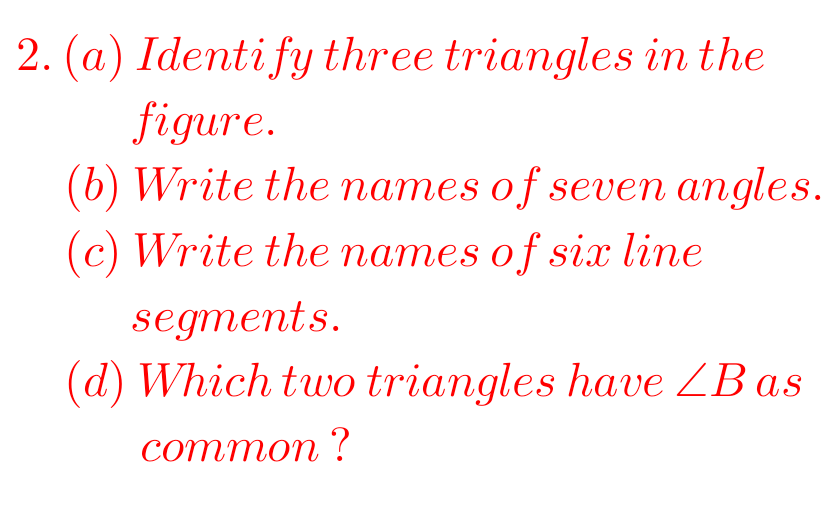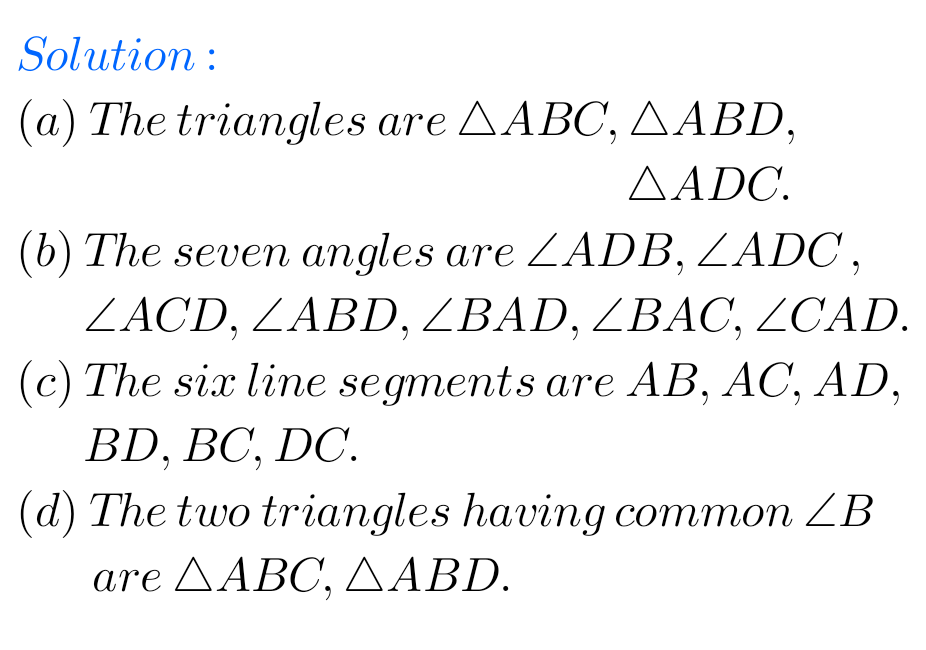## Maths Ncert Basic Geometrical Ideas Exercise 4.4 Solutions

Ncert textbook Mathematics Class 6 Chapter 4 Basic Geometrical Ideas exercise 4.4 solutions are given.

Study the lesson Basic Geometrical Ideas in the textbook.

Observe the problems and solutions given in the textbook.

Observe the given below problems and solutions and try them in your own method.

You can see Solutions for Maths Class 6

Chapter 1 Knowing our Numbers.

Chapter 2 whole Numbers

Some chapters solutions links are given below.

M

### Ncert solutions for Basic Geometrical Ideas Exercise 4.4

Chapter 4

Basic Geometrical Ideas Class 6

Exercise 4.4## Chapter 4 Basic Geometrical Ideas Exercise 4.4 solutions class 6M

#### Exercise 4.4 solutions Basic Geometrical Ideas Maths Ncert

M

Note: Observe the solutions and try them in your own method.

Chapter 3 Playing with Numbers

Chapter 4 Basic Geometrical Ideas

Exercise 4.1

Exercise 4.2

Exercise 4.3

Exercise 4.4

Exercise 4.5

Exercise 4.6

Chapter 6 Integers

Fractions

Chapter 14 Practical Geometry

Exercise 14.1

Exercise 14.2

Exercise 14.3

Exercise 14.4

Exercise 14.5